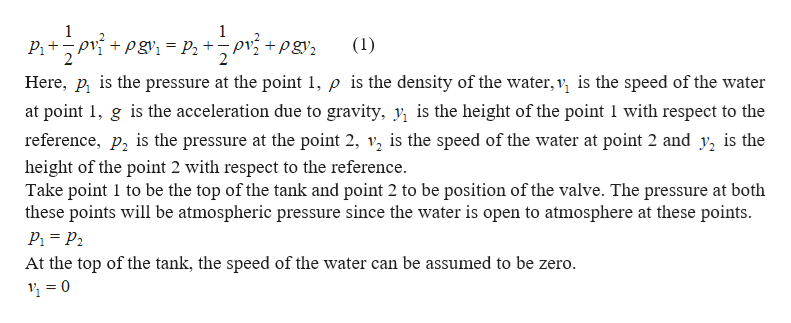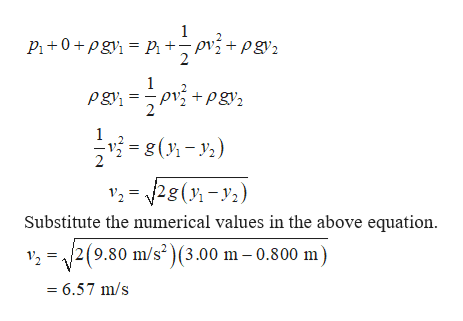# A large water tank is 3.00 m high and filled to the brim, the top of the tank open to the air. A small pipe with a faucet is attached to the side of the tank, 0.800 m above the ground. If the valve is opened, at what speed will water come out of the pipe?

Question
10 views

A large water tank is 3.00 m high and filled to the brim, the top of the tank open to the air. A small pipe with a faucet is attached to the side of the tank, 0.800 m above the ground. If the valve is opened, at what speed will water come out of the pipe?

check_circle

Step 1

The Bernoulli’s principle is given by,help_outlineImage Transcriptionclose+Pgyi = P2 + pv +pgy, (1) Here, p, is the pressure at the point 1, p is the density of the water, v, is the speed of the water at point 1, g is the acceleration due to gravity, y, is the height of the point 1 with respect to the reference, p, is the pressure at the point 2, v, is the speed of the water at point 2 and y, is the height of the point 2 with respect to the reference. Take point 1 to be the top of the tank and point 2 to be position of the valve. The pressure at both these points will be atmospheric pressure since the water is open to atmosphere at these points. P1 = P2 At the top of the tank, the speed of the water can be assumed to be zero. 1 = 0 fullscreen
Step 2

Put the above two equation...help_outlineImage TranscriptioncloseP +0 + pgy = P + + pgy2 pvý +pgy2 = 8(); - y.) v; = 28(); – y2) Substitute the numerical values in the above equation. =/2(9.80 m/s? )(3.00 m – 0.800 m) = 6.57 m/s fullscreen

### Want to see the full answer?

See Solution

#### Want to see this answer and more?

Solutions are written by subject experts who are available 24/7. Questions are typically answered within 1 hour.*

See Solution
*Response times may vary by subject and question.
Tagged in

### Physics